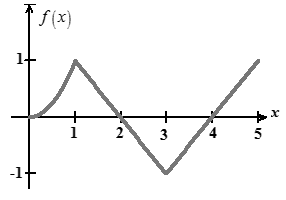# The graph of the function.### Single Variable Calculus: Concepts...

4th Edition
James Stewart
Publisher: Cengage Learning
ISBN: 9781337687805### Single Variable Calculus: Concepts...

4th Edition
James Stewart
Publisher: Cengage Learning
ISBN: 9781337687805

#### Solutions

Chapter 4, Problem 60RE
To determine

## To find: The graph of the function.

Expert Solution

The graph of the function is shown Figure-1.

### Explanation of Solution

Given information:

The function is even such that f(0)=0,f(0)=0 If 0<1,f(x)=1 if 1<x<3, and f(x)=1 if x>3 .

Calculation:

Consider f is continuous and even f(0)=0

f(x)={2x,0<x<11,1<x<31,x>3

Need to draw the graph of f(x)

Given f(x)={2x,0<x<11,1<x<31,x>3

And f(0)=0

That is function is increasing in the interval (0,1)(3,)

And decreasing in the interval (1,3)

Hence there will be a cusp at x=1,x=3

The graph of the function with above characteristics as shown Figure-1Figure-1

### Have a homework question?

Subscribe to bartleby learn! Ask subject matter experts 30 homework questions each month. Plus, you’ll have access to millions of step-by-step textbook answers!